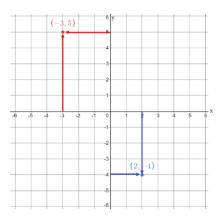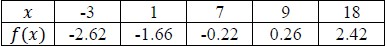# MA.912.AR.2.4Export Print
Given a table, equation or written description of a linear function, graph that function, and determine and interpret its key features.

### Clarifications

Clarification 1: Key features are limited to domain, range, intercepts and rate of change.

Clarification 2: Instruction includes the use of standard form, slope-intercept form and point-slope form.

Clarification 3: Instruction includes cases where one variable has a coefficient of zero.

Clarification 4: Instruction includes representing the domain and range with inequality notation, interval notation or set-builder notation.

Clarification 5: Within the Algebra 1 course, notations for domain and range are limited to inequality and set-builder notations.

General Information
Subject Area: Mathematics (B.E.S.T.)
Strand: Algebraic Reasoning
Status: State Board Approved

## Benchmark Instructional Guide

### Terms from the K-12 Glossary

• Coordinate Plane
• Domain
• Function Notation
• Range
• Rate of Change
• Slope
• $x$-intercept
• $y$-intercept

### Vertical Alignment

Previous Benchmarks

Next Benchmarks

### Purpose and Instructional Strategies

In grade 8, students graphed two-variable linear equations given a written description, a table, or an equation in slope-intercept form. In Algebra I, students graph linear functions from equations in other forms, as well as tables and written descriptions, and they determine and interpret the domain, range and other key features. In later courses, students will graph and solve problems involving linear programming, systems of equations in three variables and piecewise functions.
• Instruction includes representing domain and range using words, inequality notation and set-builder notation.
• Words
•  If the domain is all real numbers, it can be written as “all real numbers” or “any value of $x$, such that $x$ is a real number.”
• Inequality Notation
•  If the domain is all values of $x$ greater than 2, it can be represented as $x$ > 2.
• Set-Builder Notation
•  If the domain is all values of $x$ less than or equal to zero, it can be represented as {$x$|$x$ ≤ 0} and is read as “all values of $x$ such that $x$ is less than or equal to zero.”
• Within this benchmark, linear two-variable equations include horizontal and vertical lines. Instruction includes writing horizontal and vertical lines in the form $y$ = 3 and $x$ = −4 and as 0$x$ + 1$y$ = 3 and 1$x$ + 0$y$ = −4, respectively. Students should understand that vertical lines are not linear functions, but rather linear two-variable equations.
• Discussions about this topic are a good opportunity to foreshadow the use of horizontal and vertical lines as common constraints in systems of equations or inequalities.
• Instruction includes the use of $x$-$y$ notation and function notation.
• Instruction includes the use of appropriately scaled coordinate planes, including the use of breaks in the $x$- or $y$-axis when necessary.

### Common Misconceptions or Errors

• Students may express initial confusion with the meaning of $f$($x$) for functions written in function notation.

### Strategies to Support Tiered Instruction

• Teacher provides equations in both function notation and $x$-$y$ notation written in slope - intercept form and models graphing both forms using a graphing tool or graphing software (MTR.2.1).
• For example, $f$($x$) = $\frac{\text{2}}{\text{3}}$$x$ + 6 and y = $\frac{\text{2}}{\text{3}}$$x$ + 6, to show that both f(x) and y represent the same outputs of the function.
• Teacher provides instruction using a coordinate plane geoboard to provide students support in graphing a linear function.
• For example, given the $y$-intercept and slope of line, teacher first puts a peg at the $y$-intercept. Then, the teacher models using the slope to graph a second point by moving a second peg from the $y$-intercept “up and over” (or “down and over”) to another point. Students can check their second point by plugging it into the given equation of the line. If the equation makes a true statement, then the student has graphed the second point correctly. If the equation makes a false statement, then the student has not graphed the second point correctly.
• For students who need extra support in plotting points, teacher provides instruction using a coordinate plane geoboard to find the $x$-value on the $x$-axis and the $y$-value on the $y$- axis. Then, the teacher models moving to find the coordinate point, where the $x$- and $y$- value meet.
•  The graph below shows how a teacher could model plotting the points (−3,5) and (2, −4).• There are a total of 549 seniors graduating this year. The seniors walk across the stage at a rate of 42 seniors every 30 minutes. The ceremony also includes speaking and music that lasts a total of 25 minutes.
• Part A. Write a function that models this situation.
• Part B. What is the slope of the function you created? How does that translate to this situation?
• Part C. Graph the function you created in Part A. What is the feasible domain and range for this situation?
• Part D. For how many hours will the graduation ceremony take place?

### Instructional Items

Instructional Item 1
• Part A. Graph the function $g$($x$) = −3.6$x$ + 7.
• Part B. Identify the domain, range and $x$- and $y$-intercepts of the function.

Instructional Item 2

• Graph the linear function represented in the table below.*The strategies, tasks and items included in the B1G-M are examples and should not be considered comprehensive.

## Related Courses

This benchmark is part of these courses.
1200310: Algebra 1 (Specifically in versions: 2014 - 2015, 2015 - 2022, 2022 and beyond (current))
1200320: Algebra 1 Honors (Specifically in versions: 2014 - 2015, 2015 - 2022, 2022 and beyond (current))
1200370: Algebra 1-A (Specifically in versions: 2014 - 2015, 2015 - 2022, 2022 and beyond (current))
1200400: Foundational Skills in Mathematics 9-12 (Specifically in versions: 2014 - 2015, 2015 - 2022, 2022 and beyond (current))
7912080: Access Algebra 1A (Specifically in versions: 2014 - 2015, 2015 - 2018, 2018 - 2019, 2019 - 2022, 2022 and beyond (current))
1200315: Algebra 1 for Credit Recovery (Specifically in versions: 2014 - 2015, 2015 - 2022, 2022 and beyond (current))
1200375: Algebra 1-A for Credit Recovery (Specifically in versions: 2014 - 2015, 2015 - 2022, 2022 and beyond (current))
7912075: Access Algebra 1 (Specifically in versions: 2014 - 2015, 2015 - 2018, 2018 - 2019, 2019 - 2022, 2022 and beyond (current))
1200710: Mathematics for College Algebra (Specifically in versions: 2022 and beyond (current))
1209315: Mathematics for ACT and SAT (Specifically in versions: 2022 and beyond (current))

## Related Access Points

Alternate version of this benchmark for students with significant cognitive disabilities.
MA.912.AR.2.AP.4: Given a table, equation or written description of a linear function, select a graph of that function and determine at least two key features (can include domain, range, y-intercept or slope).

## Related Resources

Vetted resources educators can use to teach the concepts and skills in this benchmark.

## Formative Assessments

Graphing a Linear Function:

Students are asked to graph a linear function and to find the intercepts of the function as well as the maximum and minimum of the function within a given interval of the domain.

Type: Formative Assessment

Describe the Domain:

Students are given verbal descriptions of two functions and are asked to describe an appropriate domain for each.

Type: Formative Assessment

Height vs. Shoe Size:

Students are asked to identify and describe the domains of two functions given their graphs.

Type: Formative Assessment

## Lesson Plans

The Gumball Roll Lab:

This lesson is on motion of objects. Students will learn what factors affect the speed of an object through experimentation with gumballs rolling down an incline. The students will collect data through experimenting, create graphs from the data, interpret the slope of the graphs and create equations of lines from data points and the graph. They will understand the relationship of speed and velocity and be able to relate the velocity formula to the slope intercept form of the equation of a line.

Type: Lesson Plan

Whose Line Is It Anyway?:

In this lesson, students will use graphing calculators to explore linear equations in the form y = mx + b. They will observe the graphs of equations with different values of slope and y-intercept. They will draw conclusions about how the value of slope and y-intercept are visible in the appearance of the graph.

Type: Lesson Plan

Which Function?:

This activity has students apply their knowledge to distinguish between numerical data that can be modeled in linear or exponential forms. Students will create mathematical models (graph, equation) that represent the data and compare these models in terms of the information they show and their limitations. Students will use the models to compute additional information to predict future outcomes and make conjectures based on these predictions.

Type: Lesson Plan

Graphing vs. Substitution. Which would you choose?:

Students will solve multiple systems of equations using two methods: graphing and substitution. This will help students to make a connection between the two methods and realize that they will indeed get the same solution graphically and algebraically.  Students will compare the two methods and think about ways to decide which method to use for a particular problem. This lesson connects prior instruction on solving systems of equations graphically with using algebraic methods to solve systems of equations.

Type: Lesson Plan

Determining the Hubble Constant:

Students will graph distance/velocity data of real galaxies to arrive at their own value of the Hubble constant (H). Once they have calculated their own value of H, they will use it to determine distances to real galaxies with known recessional velocities.

Type: Lesson Plan

## Original Student Tutorial

Linear Functions: Jobs:

Learn how to interpret key features of linear functions and translate between representations of linear functions through exploring jobs for teenagers in this interactive tutorial.

Type: Original Student Tutorial

## MFAS Formative Assessments

Describe the Domain:

Students are given verbal descriptions of two functions and are asked to describe an appropriate domain for each.

Graphing a Linear Function:

Students are asked to graph a linear function and to find the intercepts of the function as well as the maximum and minimum of the function within a given interval of the domain.

Height vs. Shoe Size:

Students are asked to identify and describe the domains of two functions given their graphs.

## Original Student Tutorials Mathematics - Grades 9-12

Linear Functions: Jobs:

Learn how to interpret key features of linear functions and translate between representations of linear functions through exploring jobs for teenagers in this interactive tutorial.

## Student Resources

Vetted resources students can use to learn the concepts and skills in this benchmark.

## Original Student Tutorial

Linear Functions: Jobs:

Learn how to interpret key features of linear functions and translate between representations of linear functions through exploring jobs for teenagers in this interactive tutorial.

Type: Original Student Tutorial

## Parent Resources

Vetted resources caregivers can use to help students learn the concepts and skills in this benchmark.